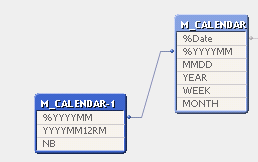QlikView App Development

Discussion Board for collaboration related to QlikView App Development.

Announcements
Highlighted
Contributor

12 rolling months

Hello community,

I'm facing an issue by trying to make a 12 rolling months in a line chart.

This chart has a calculation condition: GetSelectedCount(YEAR)=1 and GetSelectedCount(MONTH)=1

Here is my data model to make this chart :NB is field which counts the number of months different of the period YYYYMM12RM. For example :

%YYYYMM     YYYYMM12RM     NB

201404            201404                  1

201404            201403                  2

201404            201402                  3

201404            201401                  4

201404            201312                  5

201404            201311                  6

201404            201310                  7

201404            201309                  8

201404            201308                  9

201404            201307                  10

201404            201306                  11

201404            201305                  12

I use in the dimensions the field %YYYYMM.

The expression used is: Sum({<YEAR=, MONTH=, %YYYYMM={">=\$(vPeriodMin)<=\$(vPeriodMax)"}>} Sales)

where vPeriodMin=Max({<NB={'12'}>} YYYYMM12RM) and vPeriodMax=Max({<NB={'1'}>} YYYYMM12RM).

It seems that my expression doesn't take into account my Set Analysis.

Does anyone have an idea please ?

Regards,

Quentin

Tags (7)
1 Solution

Accepted Solutions
Contributor

Re: 12 rolling months

Hello,

I finally found a solution for my 12 rolling months line chart.

Here is my dimension:

=if(right(YYYYMM12RM, 2)='01',

if(YYYYMM12RM+11>=\$(vPeriodMin) and YYYYMM12RM+11<=\$(vPeriodMax),

YYYYMM12RM+11

),

if(YYYYMM12RM+99>=\$(vPeriodMin) and YYYYMM12RM+99<=\$(vPeriodMax),

YYYYMM12RM+99

)

)

Here is my expression:

Above(Sum({<YEAR=, MONTH=, %Date=, %YYYYMM={'>=\$(vPeriodMin))<=\$(vPeriodMax)'}>} [Sales Quantity MTH]), 0, 11)

Thanks to mphekin12 and Gysbert Wassenaar for their help.

Regards,

Quentin

4 Replies
Valued Contributor

Re: 12 rolling months

Quentin,

Please read this post by Gysbert Wassenaar:

Contributor

Re: 12 rolling months

Thanks for the fast answer mphekin12.

I tried to understand Gysbert's example.

In his example, the table AsOfMonth looks like my table Calendar-1. If I understood well, I just needed to change the dimension in my chart as it is showed in Gybert's example but the sum are calculated only for some date.

Regards,

Quentin

Contributor

Any ideas ?

Quentin

Contributor

Re: 12 rolling months

Hello,

I finally found a solution for my 12 rolling months line chart.

Here is my dimension:

=if(right(YYYYMM12RM, 2)='01',

if(YYYYMM12RM+11>=\$(vPeriodMin) and YYYYMM12RM+11<=\$(vPeriodMax),

YYYYMM12RM+11

),

if(YYYYMM12RM+99>=\$(vPeriodMin) and YYYYMM12RM+99<=\$(vPeriodMax),

YYYYMM12RM+99

)

)

Here is my expression:

Above(Sum({<YEAR=, MONTH=, %Date=, %YYYYMM={'>=\$(vPeriodMin))<=\$(vPeriodMax)'}>} [Sales Quantity MTH]), 0, 11)

Thanks to mphekin12 and Gysbert Wassenaar for their help.

Regards,

Quentin